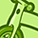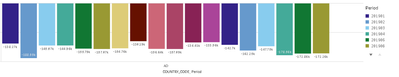# QlikView App Dev

Discussion Board for collaboration related to QlikView App Development.

Announcements
Join this live chat April 6, 10AM EST - QlikView to Qlik Sense REGISTER
cancel
Showing results for
Did you mean:Contributor III

## Qlik Above function in Bar chart not working

Hi,

I have a table like below,Each period holds YTD values (except 201901 & 202001). i wish to find out monthly figures for each period.

The same has been successfully found by using below expression .

IF((LEFT(Period,4) <> Above(LEFT(Period,4))),(SUM({<TYPE={'REEL'}>}YTD_Value)),
(SUM({<TYPE={'REEL'}>}YTD_Value)- Above(SUM({<TYPE={'REEL'}>}YTD_Value))))

Now all i need is adding additional country dimension to show  same in BAR Chart.

But when i reuse the same expression its not showing monthly value instead it shows YTD Value If i not consider period column.Any suggestion how to fix this in Bar chart to show cumulative  sum in bar chart country wise.

Labels (6)

• ### Qlik Sense Data Architect

1 Solution

Accepted SolutionsContributor III
Author

Thank you very much for your assistance.

I have fixed this case from back end scripting.

Thank You!

5 RepliesMVP

Try this

``````Aggr(

If(Left(Period, 4) <> Above(Left(Period, 4)),
Sum({<TYPE = {'REEL'}>} YTD_Value),
Sum({<TYPE = {'REEL'}>} YTD_Value) - Above(Sum({<TYPE = {'REEL'}>} YTD_Value))
)

, COUNTRY_CODE, (Period, (NUMERIC)))``````Contributor III
Author

However given expression works well when i consider period dimension in Bar Chart. Any other suggestions to show the aggregation at Country Code level.MVP

Try this may be

``````Sum(Aggr(

If(Left(Period, 4) <> Above(Left(Period, 4)),
Sum({<TYPE = {'REEL'}>} YTD_Value),
Sum({<TYPE = {'REEL'}>} YTD_Value) - Above(Sum({<TYPE = {'REEL'}>} YTD_Value))
)

, COUNTRY_CODE, (Period, (NUMERIC))))``````Contributor III
Author

Thank you very much for your assistance.

I have fixed this case from back end scripting.

Thank You!MVP

Awesome